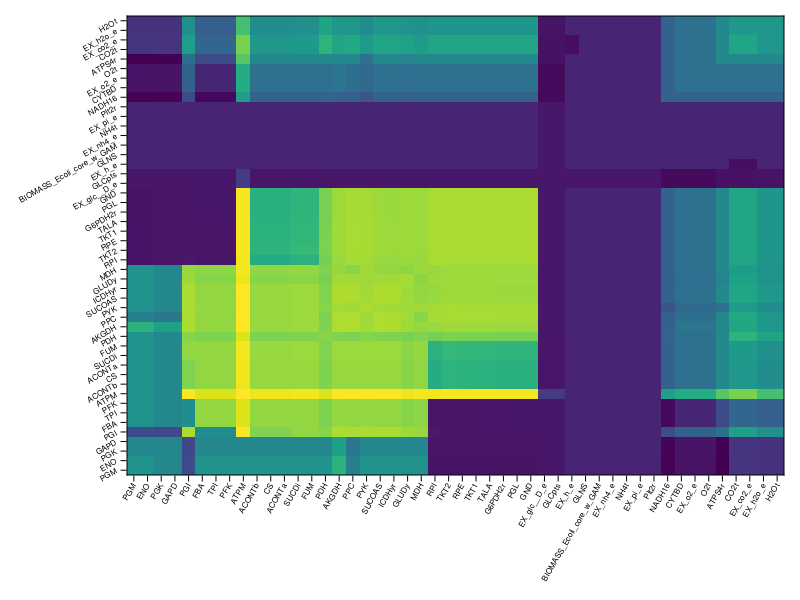# Restricting and disabling individual reactions

Here, we show several methods how to explore the effect of disabling or choking the reactions in the models.

!isfile("e_coli_core.xml") &&

using COBREXA, GLPK

model = load_model(StandardModel, "e_coli_core.json")
Metabolic model of type StandardModel
sparse([9, 51, 55, 64, 65, 34, 44, 59, 66, 64  …  20, 22, 23, 25, 16, 17, 34, 44, 57, 59], [1, 1, 1, 1, 1, 2, 2, 2, 2, 3  …  93, 93, 94, 94, 95, 95, 95, 95, 95, 95], [1.0, 1.0, -1.0, -1.0, 1.0, -1.0, 1.0, -1.0, 1.0, 1.0  …  1.0, -1.0, 1.0, -1.0, -1.0, 1.0, -1.0, 1.0, 1.0, -1.0], 72, 95)
Number of reactions: 95
Number of metabolites: 72


## Disabling a reaction

There are several possible ways to disable a certain reaction in the model. The easiest way is to use change_bound or change_bounds to create a variant of the model that has the corresponding bounds modified (or, alternatively, a pipeable "variant" version with_changed_bound).

Alternatively, you could utilize change_constraint as a modification that acts directly on the JuMP optimization model. That may be useful if you first apply some kind of complicated constraint scheme modification, such as add_loopless_constraints.

In turn, in the simple case, the following 2 ways of disabling the FBA reaction are equivalent. The first, making a variant of the model structure, might be slightly preferred because it better composes with other changes; the second does not compose as well but may be more efficient (and thus faster) in certain situations:

flux1 = flux_balance_analysis_vec(
model |> with_changed_bound("FBA", lower = 0.0, upper = 0.0),
GLPK.Optimizer,
);

flux2 = flux_balance_analysis_vec(
model,
GLPK.Optimizer,
modifications = [change_constraint("FBA", lb = 0.0, ub = 0.0)],
);

The solutions should not differ a lot:

sum((flux1 .- flux2) .^ 2)
0.0

## Restricting a reaction

Quite naturally, you can restruct the reaction to a limited flow, simulating e.g. nutrient deficiency:

original_flux = flux_balance_analysis_dict(model, GLPK.Optimizer);

restricted_flux = flux_balance_analysis_dict(
model,
GLPK.Optimizer,
modifications = [change_constraint("EX_o2_e", lb = -0.1, ub = 0.0)],
);

The growth in the restricted case is, expectably, lower than the original one:

original_flux["BIOMASS_Ecoli_core_w_GAM"], restricted_flux["BIOMASS_Ecoli_core_w_GAM"]
(0.8739215069684168, 0.21526265976482833)

## Screening for sensitive and critical reactions

Using higher-order analysis scheduling functions (in particular screen), you can easily determine which reactions play a crucial role for the model viability and which are not very important.

We can take all reactions where the flux is not zero:

running_reactions = [(rid, x) for (rid, x) in original_flux if abs(x) > 1e-3]
48-element Vector{Tuple{String, Float64}}:
("PDH", 9.282532599166663)
("PYK", 1.7581774441067894)
("CO2t", -22.809833310205036)
("EX_nh4_e", -4.765319193197479)
("CS", 6.00724957535039)
("PGM", -14.716139568742843)
("TKT1", 1.4969837572615392)
("ACONTa", 6.00724957535039)
("EX_pi_e", -3.2148950476847578)
("GLNS", 0.2234617293318454)
⋮
("PFK", 7.477381962160301)
("MDH", 5.064375661482134)
("PGI", 4.860861146496889)
("O2t", 21.799492655998836)
("EX_co2_e", 22.809833310205036)
("GND", 4.959984944574597)
("SUCDi", 5.064375661482134)
("ENO", 14.716139568742843)
("FUM", 5.0643756614821305)

...and choke these reactions to half that flux, computing the relative loss of the biomass production::

screen(
model,
variants = [
[with_changed_bound(rid, lower = -0.5 * abs(x), upper = 0.5 * abs(x))] for
(rid, x) in running_reactions
],
args = running_reactions,
analysis = (m, rid, _) ->
rid =>
flux_balance_analysis_dict(m, GLPK.Optimizer)["BIOMASS_Ecoli_core_w_GAM"] /
original_flux["BIOMASS_Ecoli_core_w_GAM"],
)
48-element Vector{Pair{String, Float64}}:
"PDH" => 0.9572568763607445
"PYK" => 0.9948533770213798
"CO2t" => 0.8143486062337915
"EX_nh4_e" => 0.500000000000007
"CS" => 0.9748219246083657
"PGM" => 0.7623549285987249
"TKT1" => 0.9947579089976174
"ACONTa" => 0.9748219246083657
"EX_pi_e" => 0.5000000000000041
"GLNS" => 0.5000000000000046
⋮
"PFK" => 0.9706579451719946
"MDH" => 0.978609337882475
"PGI" => 0.9938427223302222
"O2t" => 0.6731603824513879
"EX_co2_e" => 0.8143486062337915
"GND" => 0.9942167589515672
"SUCDi" => 0.978604672558648
"ENO" => 0.7623549285987249
"FUM" => 0.9786046725586398

(You may notice that restricting the ATP maintenance pseudo-reaction (ATPM) had a mildly surprising effect of actually increasing the biomass production by a few percent. That is because the cells are not required to produce ATP to survive and may invest the nutrients and energy elsewhere.)

## Screening with reaction combinations

The same analysis can be scaled up to screen for combinations of critical reactions, giving possibly more insight into the redundancies in the model:

running_reaction_combinations = [
(rid1, rid2, x1, x2) for (rid1, x1) in running_reactions,
(rid2, x2) in running_reactions
]

biomass_mtx = screen(
model,
variants = [
[
with_changed_bound(rid1, lower = -0.5 * abs(x1), upper = 0.5 * abs(x1)),
with_changed_bound(rid2, lower = -0.5 * abs(x2), upper = 0.5 * abs(x2)),
] for (rid1, rid2, x1, x2) in running_reaction_combinations
],
analysis = m ->
flux_balance_analysis_dict(m, GLPK.Optimizer)["BIOMASS_Ecoli_core_w_GAM"] /
original_flux["BIOMASS_Ecoli_core_w_GAM"],
)
48×48 Matrix{Float64}:
0.957257  0.958697  0.850001  0.5  0.949714  …  0.951869  0.762355  0.951869
0.958697  0.994853  0.7844    0.5  0.974822     0.978605  0.762355  0.978605
0.850001  0.7844    0.814349  0.5  0.776481     0.780017  0.533619  0.780017
0.5       0.5       0.5       0.5  0.5          0.5       0.5       0.5
0.949714  0.974822  0.776481  0.5  0.974822     0.974822  0.762355  0.974822
0.762355  0.762355  0.533619  0.5  0.762355  …  0.762355  0.762355  0.762355
0.945216  0.99001   0.811828  0.5  0.842536     0.855916  0.468124  0.855916
0.949714  0.974822  0.776481  0.5  0.974822     0.974822  0.762355  0.974822
0.5       0.5       0.5       0.5  0.5          0.5       0.5       0.5
0.5       0.5       0.5       0.5  0.5          0.5       0.5       0.5
⋮                                            ⋱  ⋮
0.948957  0.971409  0.643779  0.5  0.970658     0.970658  0.762355  0.970658
0.951869  0.984571  0.787974  0.5  0.968204  …  0.972111  0.762355  0.972111
0.957257  0.993152  0.794556  0.5  0.951246     0.967947  0.581237  0.967947
0.67316   0.649196  0.67316   0.5  0.67316      0.67316   0.467999  0.67316
0.850001  0.7844    0.814349  0.5  0.776481     0.780017  0.533619  0.780017
0.944056  0.989245  0.81164   0.5  0.839859     0.853028  0.468405  0.853028
0.951869  0.978605  0.780017  0.5  0.974822  …  0.978605  0.762355  0.978605
0.762355  0.762355  0.533619  0.5  0.762355     0.762355  0.762355  0.762355
0.951869  0.978605  0.780017  0.5  0.974822     0.978605  0.762355  0.978605

Finally, let's plot the result:

using CairoMakie, Clustering

order =
hclust([
sum((i .- j) .^ 2) for i in eachcol(biomass_mtx), j in eachcol(biomass_mtx)
]).order

labels = first.(running_reactions)[order];
positions = collect(eachindex(labels))

f = Figure(fontsize = 8)
ax = Axis(f[1, 1], xticks = (positions, labels), yticks = (positions, labels))
heatmap!(ax, positions, positions, biomass_mtx[order, order])
ax.xticklabelrotation = π / 3
ax.xticklabelalign = (:right, :center)
ax.yticklabelrotation = π / 6
ax.yticklabelalign = (:right, :center)
fRemember that screen can be parallelized just by supplying worker IDs. Use that to gain significant speedup with analyses of larger models.